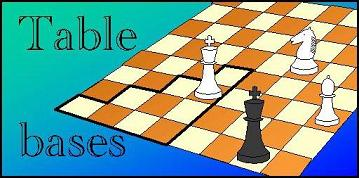Leapfrog: Index Transformation# Symmetry: mapping the Index

### Bit Operations on the Index

On the a sequence of numbers 0-7, reflecting it (i.e. reading it back to front) has the same effect as complementing the 3 bits of its binary representation: 7 = 111 <-> 0 = 000, 6 = 110 <-> 1 = 001, etc. If the squares of the chess board are numbered in the canonical order, where the 3 low-order bits indicate the file, and the next 3 bits the rank, the operation V maps each square on a square that has the low-order 3 bits flipped (which we can get by XOR-ing with 7), while H corresponds to flipping the next 3 bits (XOR-ing with 56, = 111000 in binary or 070 in octal notation). the operation D is more difficult: here we have to swap the file and rank bits. Tere is no single instruction that does this; we would have to use masks and shifts, to first separate rank and file bits, shift them to their new position, and OR them together to obtain the new square number. As the total index is just all square numbers of the pieces strung together, we can get the index for a transformed position by applying the operations described above on al square numbers that make up the index simultaneously: XOR with 07070707 (V) or 070707070 (H), or AND with these two masks (given in the octal number system!), and shift them 3 bits left or right before recombining for D.

The Leapfrog generator will assign a symmetry operation to each position (dependent only on the board location of the first two (white) man in that position). This is the operation that will have to be applied on the position in order to get the equivalent canonical representative, which is stored in the tablebase. For any of the 4096 possible locations of the first two pieces this will be tabulated in a 64x64 table.